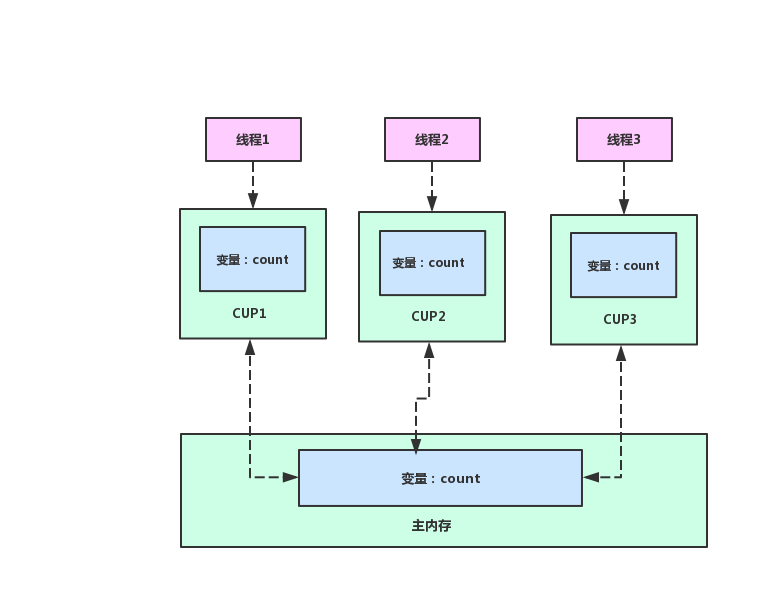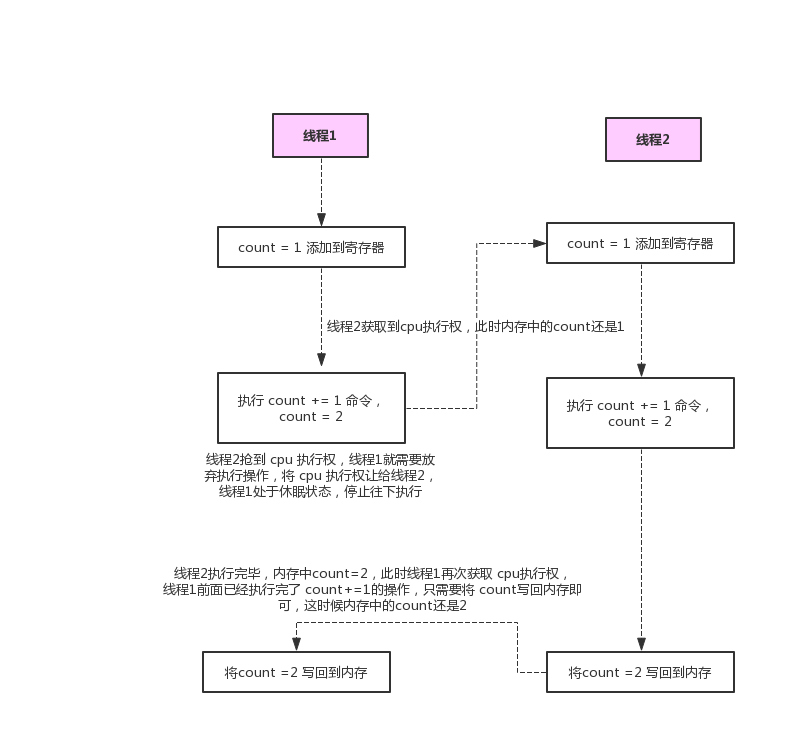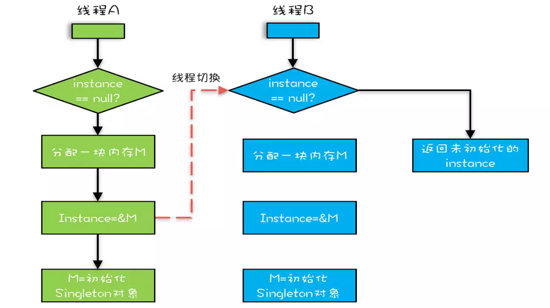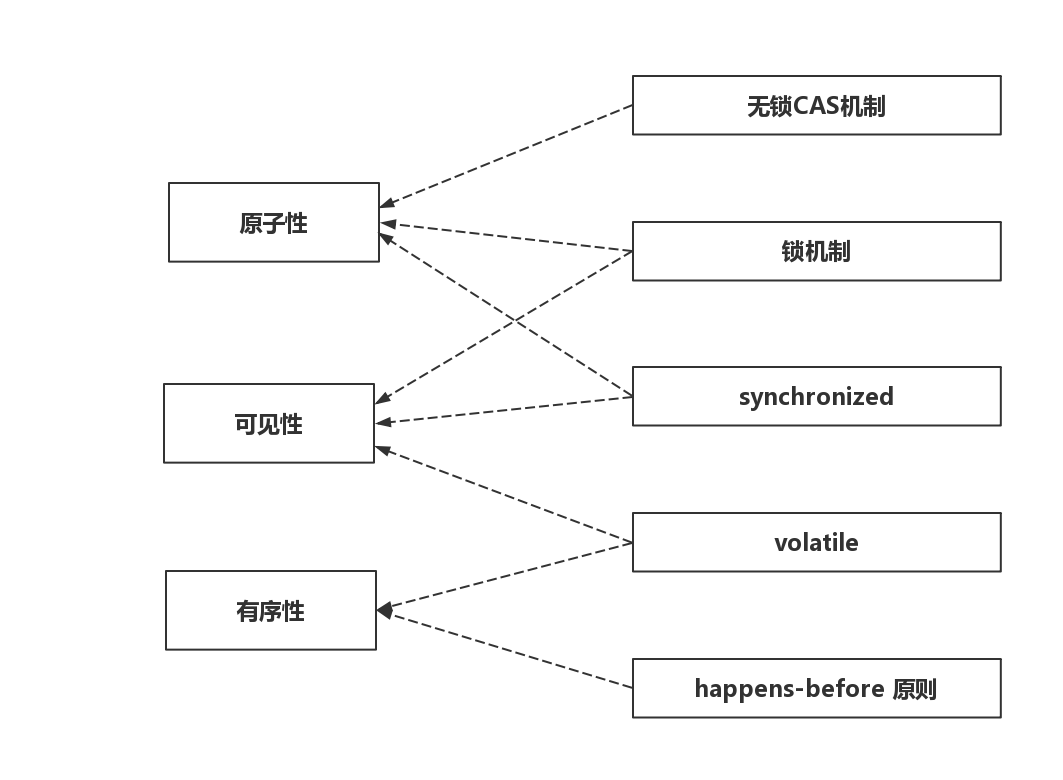# 你的 Java 并发程序 Bug，100% 是这几个原因造成的

## 可见性问题```public class Demo {
private int count = 0;
// 每个线程为count + 10000
for (int i = 0; i < 10000; i++) {
count += 1;
}
}
public static void main(String[] args) throws InterruptedException {
for (int i = 0; i < 10; i++) {
Demo demo = new Demo();
});
});
t1.start();
t2.start();
t1.join();
t2.join();
System.out.println(demo.count);
}
}
}
```

## 原子性问题

• 把变量 count 从内存加载到 CPU 的寄存器，假设 count = 1
• 在寄存器中执行 count +1 操作，count = 1+1 =2
• 将结果 +1 后的 count 写入内存## 有序性问题

```1  int i = 1;
2  int m = 11;
3  long x = 23L;
```

```public class Singleton {
static Singleton instance;
public static Singleton getInstance(){
// 第一次判断
if (instance == null) {
// 加锁，只有一个线程能够获取锁
synchronized(Singleton.class) {
// 第二次判断
if (instance == null)
// 构建对象，这里面就非常有学问了
instance = new Singleton();
}
}
return instance;
}
}
```

• 指令1：分配一块内存 M；
• 指令2：在内存 M 上初始化 Singleton 对象；
• 指令3：然后 M 的地址赋值给 instance 变量。

• 指令1：分配一块内存 M；
• 指令2：将 M 的地址赋值给 instance 变量；
• 指令3：最后在内存 M 上初始化 Singleton 对象。### 最后